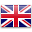English

# The model

We begin by describing the simplest version of our model. Later on, we will introduce several modifications, in order to deal with time dependent parameters.

Consider a hidden process (the non-observable actual number of counts in the phenomenon under study)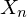with Po-INAR(1) structure:

(1)where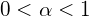is a fixed parameter,Poisson(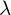), i.i.d., independent ofare the innovations, andis the binomial thinning operator: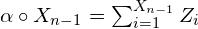withi.i.d Bernoulli(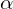) random variables. Later on, we shall introduce time dependence, and hence we will be considering thatis a funtion of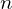.

The INAR(1) process is a homogeneous Markov chain with transition probabilitiesThe expectation and variance of the  binomial thinning operator are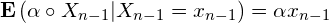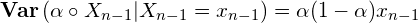A simple under-reporting scheme

The under-reported phenomenon is modeled by assuming that the observed counts are

(2)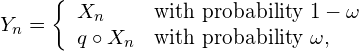where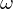andrepresent the frequency and intensity of the under-reporting process, respectively. We will eventually be interested in considering thatis time dependent:. That is, for each, we observewith probability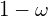, and a-thinning ofwith probability, independently of the past.

Hence, what we observe (the reported counts) areProperties of the model

The mean and the variance of a stationary INAR(1) processwith Poisson() innovations are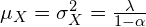.
Its auto-covariance and auto-correlation functions are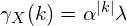andrespectively.
Hence,

(3)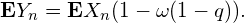The auto-covariance function of the observed process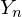is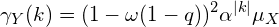Hence, the auto-correlation function ofis a multiple of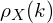:Parameter estimation

The marginal probability distribution ofis a \textbf{mixture of two Poisson distributions}

(4)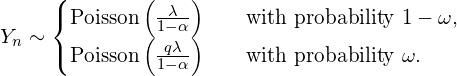Whenthe distribution of the observed process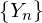is a zero-inflated Poisson distribution.

From the mixture we derive initial estimations for,,and, to be used in a maximum likelihood estimation procedure.

The likelihood function ofis quite cumbersome to compute,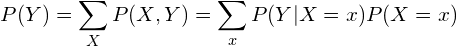hence the  forward algorithm (Lystig and Hughes (2002)),  used in the context of HMC is a suitable option.

Consider the  forward probabilities

(5)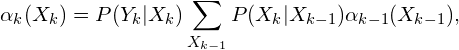with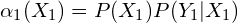.

Then, the likelihood function is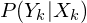andare the so-called emission and transition probabilities.

Transition probabilities are computed as

(6)While emission probabilities are given by

(7)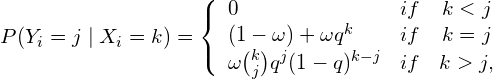From this computations, a nonlinear optimization program computes the MLE estimates of the parameters.

Reconstructing the hidden chainIn order to reconstruct the hidden series, the  Viterbi algorithm (Viterbi, 1967) is used.

The idea is to provide the latent chain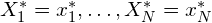that maximizes the likelyhood of the latent process given the observed series, assuming all the parameters are known.

Let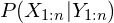be the likelihood function of the model, then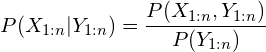Sincedoes not depend on, it is enough to maximise the probability.

The hidden series is reconstructed as:Predictions

Having observed, we are interested in predicting, for, and in evaluating the uncertainty of these predictions.

From equation 3, we have that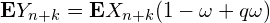, so that, if we have a good estimate for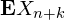, then we can predictby means of its expectation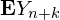.

From (1), assuming that the expectation of the innovations depends on, that is, the noise is Poisson(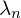) it is straightforward to see that

(8)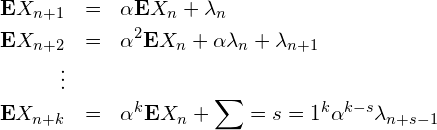The easiest way to estimate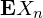is by substitutingbyin (3), to get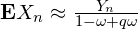, and then in 8 to get

(9)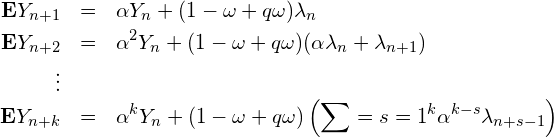Bibliography

T.C. Lystig, J.P. Hughes (2002),  Exact computation of the observed information matrix for hidden Markov models, Jr of Comp.and Graph. Stat.

Viterbi, A.J. (1967),  Error bounds for convolutional codes and an asymptotically optimum decoding algorithm. IEEE Transactions on Information Theory, 13, 260-269